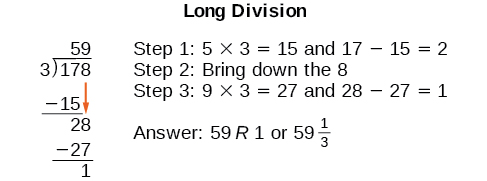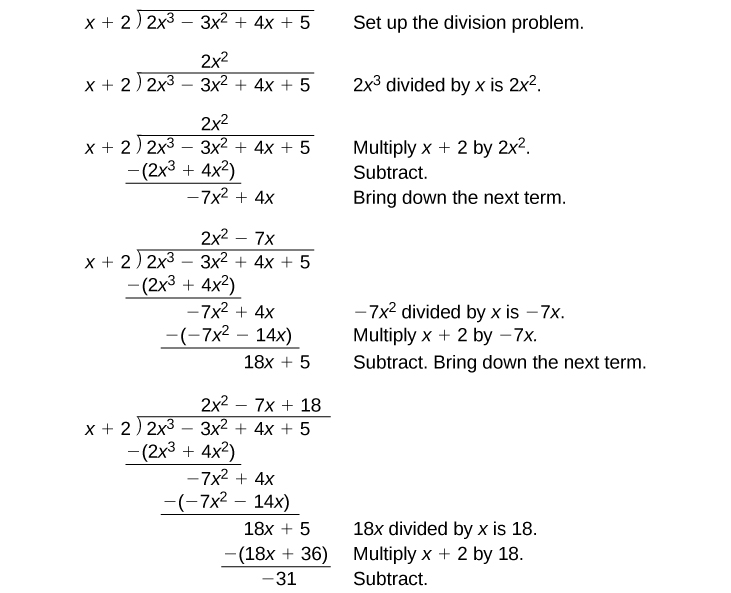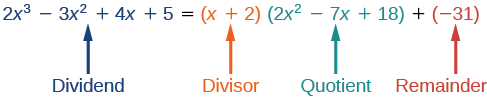# 5.4 Dividing polynomials

 Page 1 / 6
In this section, you will:
• Use long division to divide polynomials.
• Use synthetic division to divide polynomials.Lincoln Memorial, Washington, D.C. (credit: Ron Cogswell, Flickr)

The exterior of the Lincoln Memorial in Washington, D.C., is a large rectangular solid with length 61.5 meters (m), width 40 m, and height 30 m. National Park Service. "Lincoln Memorial Building Statistics." http://www.nps.gov/linc/historyculture/lincoln-memorial-building-statistics.htm. Accessed 4/3/2014 We can easily find the volume using elementary geometry.

$\begin{array}{ccc}\hfill V& =& l\cdot w\cdot h\hfill \\ & =& 61.5\cdot 40\cdot 30\hfill \\ & =& 73,800\hfill \end{array}$

So the volume is 73,800 cubic meters $\text{\hspace{0.17em}}\left(\text{m}³\right).\text{\hspace{0.17em}}$ Suppose we knew the volume, length, and width. We could divide to find the height.

$\begin{array}{ccc}\hfill h& =& \frac{V}{l\cdot w}\hfill \\ & =& \frac{73,800}{61.5\cdot 40}\hfill \\ & =& 30\hfill \end{array}$

As we can confirm from the dimensions above, the height is 30 m. We can use similar methods to find any of the missing dimensions. We can also use the same method if any, or all, of the measurements contain variable expressions. For example, suppose the volume of a rectangular solid is given by the polynomial $\text{\hspace{0.17em}}3{x}^{4}-3{x}^{3}-33{x}^{2}+54x.\text{\hspace{0.17em}}$ The length of the solid is given by $\text{\hspace{0.17em}}3x;\text{\hspace{0.17em}}$ the width is given by $\text{\hspace{0.17em}}x-2.\text{\hspace{0.17em}}$ To find the height of the solid, we can use polynomial division, which is the focus of this section.

## Using long division to divide polynomials

We are familiar with the long division algorithm for ordinary arithmetic. We begin by dividing into the digits of the dividend that have the greatest place value. We divide, multiply, subtract, include the digit in the next place value position, and repeat. For example, let’s divide 178 by 3 using long division.Another way to look at the solution is as a sum of parts. This should look familiar, since it is the same method used to check division in elementary arithmetic.

We call this the Division Algorithm and will discuss it more formally after looking at an example.

Division of polynomials that contain more than one term has similarities to long division of whole numbers. We can write a polynomial dividend as the product of the divisor and the quotient added to the remainder. The terms of the polynomial division correspond to the digits (and place values) of the whole number division. This method allows us to divide two polynomials. For example, if we were to divide $\text{\hspace{0.17em}}2{x}^{3}-3{x}^{2}+4x+5\text{\hspace{0.17em}}$ by $\text{\hspace{0.17em}}x+2\text{\hspace{0.17em}}$ using the long division algorithm, it would look like this:We have found

$\frac{2{x}^{3}-3{x}^{2}+4x+5}{x+2}=2{x}^{2}-7x+18-\frac{31}{x+2}$

or

$\frac{2{x}^{3}-3{x}^{2}+4x+5}{x+2}=\left(x+2\right)\left(2{x}^{2}-7x+18\right)-31$

We can identify the dividend , the divisor , the quotient , and the remainder .Writing the result in this manner illustrates the Division Algorithm.

## The division algorithm

The Division Algorithm    states that, given a polynomial dividend $\text{\hspace{0.17em}}f\left(x\right)\text{\hspace{0.17em}}$ and a non-zero polynomial divisor $\text{\hspace{0.17em}}d\left(x\right)\text{\hspace{0.17em}}$ where the degree of $\text{\hspace{0.17em}}d\left(x\right)\text{\hspace{0.17em}}$ is less than or equal to the degree of $\text{\hspace{0.17em}}f\left(x\right)$ , there exist unique polynomials $\text{\hspace{0.17em}}q\left(x\right)\text{\hspace{0.17em}}$ and $\text{\hspace{0.17em}}r\left(x\right)\text{\hspace{0.17em}}$ such that

$f\left(x\right)=d\left(x\right)q\left(x\right)+r\left(x\right)$

$q\left(x\right)\text{\hspace{0.17em}}$ is the quotient and $\text{\hspace{0.17em}}r\left(x\right)\text{\hspace{0.17em}}$ is the remainder. The remainder is either equal to zero or has degree strictly less than $\text{\hspace{0.17em}}d\left(x\right).\text{\hspace{0.17em}}$

If $\text{\hspace{0.17em}}r\left(x\right)=0,\text{\hspace{0.17em}}$ then $\text{\hspace{0.17em}}d\left(x\right)\text{\hspace{0.17em}}$ divides evenly into $\text{\hspace{0.17em}}f\left(x\right).\text{\hspace{0.17em}}$ This means that, in this case, both $\text{\hspace{0.17em}}d\left(x\right)\text{\hspace{0.17em}}$ and $\text{\hspace{0.17em}}q\left(x\right)\text{\hspace{0.17em}}$ are factors of $\text{\hspace{0.17em}}f\left(x\right).\text{\hspace{0.17em}}$

#### Questions & Answers

write down the polynomial function with root 1/3,2,-3 with solution
if A and B are subspaces of V prove that (A+B)/B=A/(A-B)
write down the value of each of the following in surd form a)cos(-65°) b)sin(-180°)c)tan(225°)d)tan(135°)
Prove that (sinA/1-cosA - 1-cosA/sinA) (cosA/1-sinA - 1-sinA/cosA) = 4
what is the answer to dividing negative index
In a triangle ABC prove that. (b+c)cosA+(c+a)cosB+(a+b)cisC=a+b+c.
give me the waec 2019 questions
the polar co-ordinate of the point (-1, -1)
prove the identites sin x ( 1+ tan x )+ cos x ( 1+ cot x )= sec x + cosec x
tanh`(x-iy) =A+iB, find A and B
B=Ai-itan(hx-hiy)
Rukmini
what is the addition of 101011 with 101010
If those numbers are binary, it's 1010101. If they are base 10, it's 202021.
Jack
extra power 4 minus 5 x cube + 7 x square minus 5 x + 1 equal to zero
the gradient function of a curve is 2x+4 and the curve passes through point (1,4) find the equation of the curve
1+cos²A/cos²A=2cosec²A-1
test for convergence the series 1+x/2+2!/9x3By